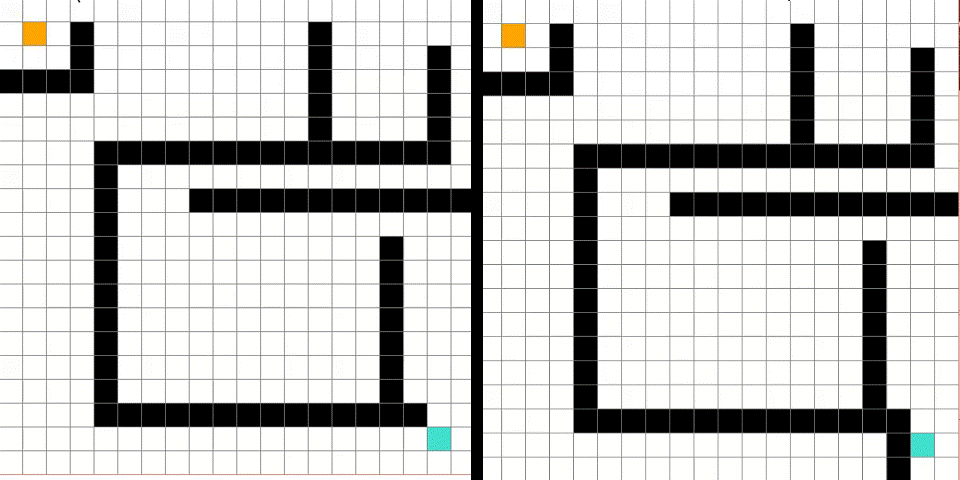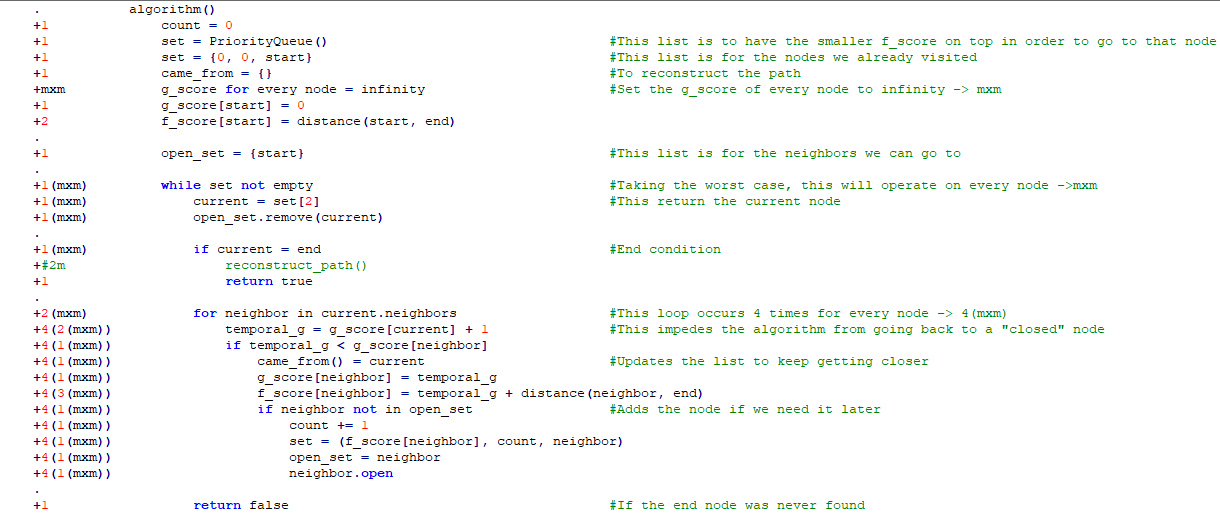# A* Star Pathfinding

When we talk about pathfinding, we usually think of an app that can show us the route to a certain place, and this apps certainly have to deal with many things, not only with all the paths that can lead us to our destiny, but also external factors like traffic and closed streets, after all the variables have been analyzed, we get a route and that´s it.

In the background, it’s actually more complicated than that. At the start, the algorithm may not have an idea of what the space looks like so it has to look almost everywhere hoping that eventually it finds the goal, this is the case for uninformed search algorithms.

A* Star algorithm is an informed search algorithm, so it has an idea of where the goal is using a function called “Heuristic”, with the function’s help, it reduces the search time. A* is based on Dijkstra’s algorithm but uses the heuristic to be faster.

## Algorithm and Time complexity.

The A* star algorithm can be used as a feature in many places that will be discussed later. But the algorithm alone needs a way to be interpreted, that´s why a GUI was needed.

But for now, let’s ignore the GUI, we are going to focus exclusively on the algorithm and use some pseudocode to try to find out the time complexity. The only problem is that the time complexity of informed algorithms are based on the heuristic function. So let´s try to do this knowing that using the worst possible heuristic function results in the algorithm having an exponential time O(cⁿ). And using a better heuristic it improves to a polynomial time O(nᶜ).

We are going to assume that the size of the grid is m*m nodes.

This first part is to verify the state of the neighbors of every node, the information can be used in the algorithm() function.

Before going into the function, there are some variables that may need some explanation to understand them better. The variables are G score and F score.

• The G score is the distance the algorithm has traveled since the start node, every node has a distance 1 from each other, so a smaller G score means a smaller path.
• The F score is the heuristic function value, this function is similar to a distance formula from one node to the end node, the smaller the F score is, the closer we are.On the right we are tricking the algorithm as it approaches the end node through a dead end.

This F score is very useful because it narrows the amount of nodes we have to look unless it is absolutely necessary because it can also be “tricked” into thinking it is geting closer, like in the example.

Now let´s check what happens inside the A* algorithm.Operations count on the left of the code, and some notes on the right.

When we count this operations, there´s no way to know when is the heuristic working, it depends on the position of the start, end and barriers, so we take the most general case: when the algorithm goes to every node. And we can see that it takes a lot of operations depending on the size of the grid.

The whole code can be found on Github. To run the GUI, you’ll need the pygame package.

Now let´s see some path finding solved by the algorithm.

## Applications

Originally, the algorithm was designed as a better version of Dijkstra´s algorithm and it was implemented on a robot to let it find his own way to in a known room but there was a problem when the robot know nothing about where it was.

So it was reimagined in other places like in videogames where you already have a map and a coordinate system to support this; for example RTS (Real Time Strategy) games like Age of Empires, Starcraft, World of Warcraft and recently, it is extremely used in other indie games.